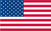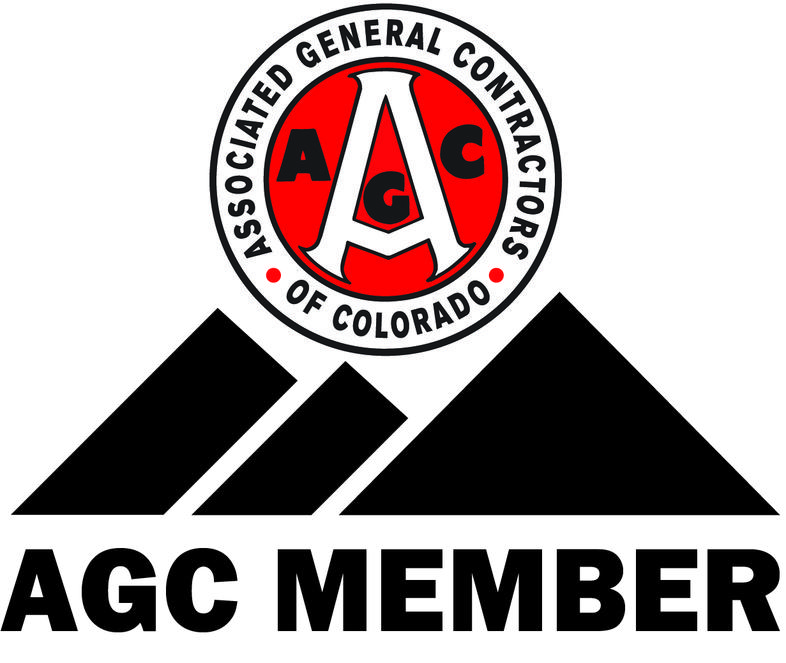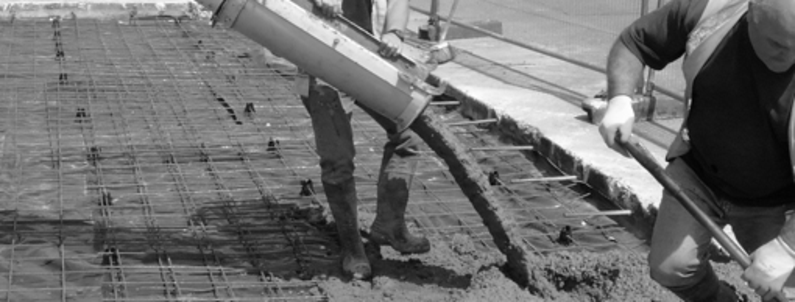HOMEABOUT USCONTACT USJOBSPHOTOSCONCRETESAND & GRAVELPRECAST CONCRETECONCRETE CALCULATORReady-mixed concrete is sold by volume measured in cubic yards. If you can determine the area of your concrete project (length x width for rectangular projects) and know the thickness of the slab, you can use the information in the Concrete Volume Calculator below to determine how much concrete you need.

One Cubic Yard of Concrete …
4" thick - covers 81 square feet
5" thick - covers 65 square feet
6" thick - covers 54 square feet
7" thick - covers 46 square feet
8" thick - covers 41 square feet
10" thick - covers 32 square feet
12" thick - covers 27 square feet

For example, let's calculate how much concrete is needed for a 5-inch thick rectangular driveway, 60-feet long (length) and 18-feet wide (width). The area of the driveway is 1,080 square feet (60-feet x 18-feet). According to the table above, one cubic yard of concrete placed at a thickness of 5-inches covers 65 square feet. In a perfect universe, our 1,080 square foot driveway will need exactly 16.62 cubic yards of concrete (1,080 square feet / 65 square feet equals 16.62 cubic yards).

But job sites are not perfect, and batch plants cannot produce concrete to such exacting volumes. To allow for spillage during placement, form movement, uneven subgrade, and consolidation, most contractors order 10% to 15% more concrete than the computed volume for the forms, and round up to the nearest quarter yard. In our example 16.62 cubic yards x 105% = 17.451 cubic yards. Rounded up to the nearest quarter yard, we would request 17.5 cubic yards of concrete when ordering concrete for the project.

For irregular-shaped pavements and slabs, create a scale drawing of the project. Divide the irregular shape into a series of regular rectangles and determine the area for each rectangle in the series. Use the table above to calculate how many cubic yards of concrete would be needed for each rectangle in the series. Add the cubic yard volumes of every rectangle in the series, multiply by 105%, and round up to the nearest quarter yard.
CONCRETE CALCULATOR...